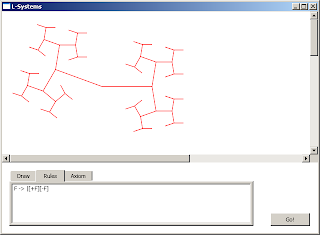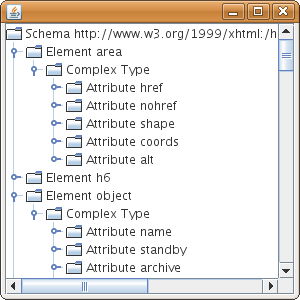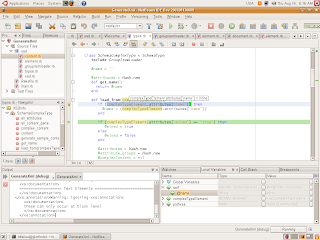## Tuesday, August 28, 2007

### Exploring L-Systems with F# and C#

In this post I'm going to show a little program for displaying graphical representations of L-Systems using turtle graphics implemented in F#, C# and WPF.

First of all this program could be easily implemented using only F#, but for me is interesting to see the interaction between native F# type/structures and C#. Because of this, the code that performs the L-system rewrite is written in F# and the code that takes the result is written in C# and uses WPF.

The first thing we need is an implementation of the turtle. Since we want to use this library with several graphics toolkits, we define our own point type for the generated data.

``#lightnamespace Langexplr.Lsystemsopen  Systemopen  Microsoft.FSharp.Math.Vector type Point =   {x : int; y : int }module Funcs = begin  let my_create_vector(i,j) =      let result = (create 2 0.0)       result. <- i      result. <- j      resultendtype TurtleGraphics =   class     val mutable direction : vector     val mutable position : Point          new(iX,iY) = {  position = {x = iX; y = iY};                       direction = Funcs.my_create_vector(1.0,0.0)}     member t.Position        with get() = t.position and             set(v) =  t.position <- {x = v.x; y = v.y }                  member t.Direction       with get() = t.direction and             set(v) =  t.direction <- v          member t.Advance(distance : int) =       let aX = int_of_float (t.direction. * float_of_int distance)       let aY = int_of_float (t.direction. * float_of_int  distance)             t.position <- { x = aX+t.position.x;                       y = aY+t.position.y }       t.position         member t.Rotate(angle) =       let nI = (t.direction. * Math.Cos(angle)) - (t.direction. * Math.Sin(angle))       let nJ = (t.direction. * Math.Sin(angle)) + (t.direction. * Math.Cos(angle))              t.direction <- Funcs.my_create_vector(nI,nJ)       end``

Now we need to represent the elements required for the L-Systems. The following elements are required:

 Start point or axiom the initial sequence of elements Rules L-system substitution rules Angle The angle used when rotating the turtle Number of iterations The number of times the rules will be applied to the axiom Size of the initial segment The size in pixels of the line that is drawn when the turtle moves forward

Also the elements inside the rule and the axiom must be translated to turtle graphics commands. The following commands are supported:

 `|` Draws a line forward, the size of the line inversely proportional to the iteration number `+` Turn left by the specified angle `-` Right left by the specified angle `[` Saves the position and direction of the turtle in a stack `]` Restores the position and direction of the turtle from the stack Letter If activated, draws a line forward

The following code shows the implementation of this:

``#lightnamespace Langexplr.Lsystemsopen Langexplr.Lsystemsopen  Systemtype LsystemElement =   | Var of String   | Constant of String      | PipeCommand of int     type Rule =   | Rule of LsystemElement * LsystemElement listmodule LsystemFuncs = begin  let rec gettingLsystemElements (str:string) i result =     if str.Length > i then       if  System.Char.IsLetterOrDigit(str.[i]) then         gettingLsystemElements str (i+1) ((Var(str.[i].ToString()))::result)       else         gettingLsystemElements str (i+1) ((Constant(str.[i].ToString()))::result)     else        List.rev result let getLsystemElements str =     gettingLsystemElements str 0 []endtype TurtleGraphicsLsystemProcessor =  class    val start : LsystemElement list    val angle : double    val rules : Rule list    val seg_size : int    val mutable saved_positions : Point list    val mutable saved_directions : vector list    val mutable drawVariables : bool        new (a_start,a_angle,t_rules,s_size,drawVars) = {        start = a_start;        angle = (Math.PI/180.0)* a_angle;        rules = t_rules;        seg_size = s_size;       saved_positions = [];       saved_directions = [];       drawVariables = drawVars}                   member lp.generate_for n current =       match n with       | 0 -> current       | o -> lp.generate_for (n - 1) (lp.apply_rules current n)        member lp.apply_rules elements iteration =       match elements with       | ((Var v)::rest) -> List.append (lp.apply_rule_for v) (lp.apply_rules rest iteration)       | ((Constant "|")::rest) -> (PipeCommand iteration)::(lp.apply_rules rest iteration)       | (e::rest) -> e::(lp.apply_rules rest iteration)       | [] -> elements        member lp.apply_rule_for v =       match (List.tryfind (fun r -> match r with                                     | Rule(Var vvar,_) when vvar = v -> true                                     | _ -> false)                     lp.rules) with       | Some (Rule(_, result)) -> result       | None -> [Var v]                 member lp.generate_iteration n (tg:TurtleGraphics)=       let final = lp.generate_for n lp.start       in           List.rev(lp.generate_points n final tg [tg.Position] [])              member lp.generate_points iterations elements (tg:TurtleGraphics) current lines =       match elements with       | ((Var _)::rest) ->              if (lp.drawVariables) then                tg.Advance(lp.seg_size)                lp.generate_points iterations rest tg (tg.Position::current) lines             else                lp.generate_points iterations rest tg current lines       | ((Constant "+")::rest) ->              tg.Rotate(lp.angle)             lp.generate_points iterations rest tg current lines       | ((Constant "-")::rest) ->              tg.Rotate(-1.0*lp.angle)             lp.generate_points iterations rest tg current lines       | ((Constant "[")::rest) ->              lp.saved_directions <- tg.Direction::lp.saved_directions             lp.saved_positions <- tg.Position::lp.saved_positions             lp.generate_points iterations rest tg current lines       | ((Constant "]")::rest) ->              match (lp.saved_directions,lp.saved_positions) with             | (cdir::rest_dir,cpos::rest_pos) ->                   lp.saved_directions <- rest_dir                  lp.saved_positions <- rest_pos                  tg.Position <- cpos                  tg.Direction <- cdir                  lp.generate_points iterations rest tg [tg.Position] (current::lines)             | _ -> lp.generate_points iterations rest tg current lines        | ((Constant "|")::rest) ->              tg.Advance(lp.seg_size / (iterations) )             lp.generate_points iterations rest tg (tg.Position::current) lines       | ((PipeCommand iteration)::rest) ->              tg.Advance(lp.seg_size / (iterations - iteration) )             lp.generate_points iterations rest tg (tg.Position::current) lines       | (_::rest) -> lp.generate_points iterations rest tg current lines       | [] -> current::lines                              end``

The `generate_iteration` method is the one that generates the line information. Its result is a list of lists of elements of type `Point`.

The C# code that takes this F# list of lists of Points and converts it to a group of Polyline instances is the following:

``private void b_Click(object sender, RoutedEventArgs e){    string origin = this.originTB.Text;    int originX = 50;    int originY = 50;    string[] oparts = origin.Split(',');    if (oparts.Length == 2)    {        originX = int.Parse(oparts);        originY = int.Parse(oparts);    }    TurtleGraphics tg = new TurtleGraphics(originX, originY);    int iterations = int.Parse(this.iterationsTB.Text);    this.canvas1.Children.Clear();    List<Rule> rules = GetRules();    TurtleGraphicsLsystemProcessor tgls =        new TurtleGraphicsLsystemProcessor(            LsystemFuncs.getLsystemElements(this.axiomTextBox.Text),            int.Parse(this.angleTB.Text),            Microsoft.FSharp.Collections.ListModule.of_IEnumerable<List<Rule>, Rule>(rules),            int.Parse(this.segmentSizeTB.Text),            drawVariablesCB.IsChecked == true);    var pointCollections =        from pc in (tgls.generate_iteration(int.Parse(this.iterationsTB.Text))).Invoke(tg)        select (new PointCollection(                  from p in pc                  select new System.Windows.Point(p.x, p.y)));    foreach (PointCollection pcol in pointCollections)    {        Polyline pLine = new Polyline();        pLine.Points = pcol;        pLine.Stroke = this.colorButton.Background;        this.canvas1.Children.Add(pLine);    }}``

What is interesting to see is that the list generated in F# is easily manipulated using LINQ. The expression that sets the value of `pointCollections` takes the native list of lists of points and converts it to a list of PointCollection objects in one expression .

Another interesting thing about the interaction between F# and C# is that the definition of the `generate_iteration` says that it could be applied with one or two arguments (because of Currying) this is used in C# by invoking the result of calling the method with one argument: `(tgls.generate_iteration(int.Parse(this.iterationsTB.Text))).Invoke(tg)`.

Executing the program with the following L-system (from Wikipedia):Also with using the "|" command:Code for this experiment can be found here.

## Friday, August 17, 2007

### Little JRuby experiment

Today I ran a Ruby program from a previous post with JRuby. I worked without modifications!

It's very nice to know that you can access Java libraries from within a Ruby. For example I decided to do a little experiment to create a TreeModel to display the contents of the loaded XSD definition in a JTree.

Here's an initial (and incomplete) implementation of the model:

``require "java"include_class 'javax.swing.JTree'include_class 'javax.swing.JFrame'include_class 'javax.swing.JScrollPane'include_class 'java.awt.BorderLayout'include_class 'javax.swing.tree.TreeModel'class XsdTreeModel   include TreeModel  attr_reader :schema  def initialize(s)    @schema = s  end  def getChild(a,i)          case a      when XSDInfo::SchemaInformation        return a.elements.values[i]                                       when XSDInfo::SchemaElement        return a.element_type      when XSDInfo::SchemaComplexType        return a.attributes.values[i]      end  end        def getChildCount(a)    case a      when XSDInfo::SchemaInformation        return a.elements.length      when XSDInfo::SchemaElement        return 1      when XSDInfo::SchemaComplexType        return a.attributes.length      else        return 0      end  end  def getIndexOfChild(a,h)     case a      when XSDInfo::SchemaInformation        return a.elements.values.index(h)      when XSDInfo::SchemaElement        return 0      when XSDInfo::SchemaComplexType        return a.attributes.index(h)      else         return -1      end  end  def getRoot()    return @schema  end  def isLeaf(o)    return false  end  # Interface methods without implementation   def addTreeModelListener(l)  end  def removeTreeModelListener(l)  end  def valueForPathChanged(arg0, arg1)   endend``

Now we can use it:

``sc = XSDInfo::SchemaCollection.newsc.add_schema XSDInfo::SchemaInformation.new("xhtml1-strict.xsd")sc.namespaces.each {|ns| sc[ns].solve_references sc}a_schema = sc[sc.namespaces]f = JFrame.newf.setSize(300,300)f.getContentPane.setLayout(BorderLayout.new)tree = JTree.newsp = JScrollPane.new(tree)tree.setModel(XsdTreeModel.new(a_schema))f.getContentPane.add(sp,BorderLayout::CENTER)f.setVisible(true)``

Running this program generates the desired tree:## Thursday, August 16, 2007

### Enjoying Netbeans Ruby integration

While listening to the Java Posse I noticed the work that Sun is doing with the Netbeans Ruby integration

This integration can be found in NetBeans IDE 6.0 Milestone 10 (M10). However, by reading Tor Norbye's blog I learned about nice features that are only available with the daily builds. So I decided to download a recent build and give it a try.

Here's some things that I found interesting and useful:

Debugger

Nice debugger integration with the IDE.Highlight block open/close element

This is a very useful feature to find out if nested blocks are closed.Code navigator

To quickly program elements inside a file.Hints

Display hints to improve the code or fix a bug. For example while editing a program from a previous post it showed me this:Also it suggest a fix for that issue:Very nice!

## Tuesday, August 14, 2007

### First steps to generate sample XML files from XSD with Ruby

As my first non-"hello world" program in Ruby I wanted to create something that was useful for me (or at least something entertaining). A couple of weeks ago I had the necessity of generating a sample file for a given XSD Schema . Eclipse already does something like this, but I thought it was a fun programming exercise.

I wanted to create something that loads the XSD Schema into a object structure that can be queried in order to the determine the elements that will be generated. I didn't look for a existing library that does this because it will be a much better exercise to try to build it myself. However creating something that support the full XSD specification like this or this is a HUGE task so I chose to support only a small subset of it.

For XML parsing and generation, I'm using REXML which is a very nice library for XML manipulation.

The basic strategy for loading the XSD Schema is to create a collection of classes that handles each part of the supported schema features. For example `SchemaElement` for supporting element declarations and `SchemaComplexType` was created for supporting the complexType declarations.

Since an XSD Schema is a common XSD document loading each element is done by using a `load_from `, for example for `SchemaElement` the `load_from` method looks like this:
``class SchemaElement   ...   def load_from(elementDefinition,prefixes)             @name = elementDefinition.attributes["name"]      if (elementDefinition.attributes["type"]) then          @element_type = Reference.new(elementDefinition.attributes["type"],prefixes)      end                if (elementDefinition.attributes["substitutionGroup"]) then          @substitution_group = Reference.new(elementDefinition.attributes["substitutionGroup"],prefixes)      end                elementDefinition.find_all {|e| !e.is_a?(REXML::Text)}.each{|e|           case e.name               when "complexType"                   ct = SchemaComplexType.new                   ct.load_from(e,prefixes)                   @element_type = ct               else                   print ""Warning: ignoring #{e}"           end      }   end...end``

As shown in the `load_from` method, there're relationships between schema elements, for example the type of the element could be a type defined elsewhere inside this schema or an imported schema. Once the schema is loaded, there's a process that takes the references and replace them with a real reference to the object. For the `SchemaElement` the `solve_references_method` looks like this:
``class SchemaElement  ...  def solve_references(collection)     if @substitution_group.is_a? (XSDInfo::Reference) then        @substitution_group = collection.get_type(                                   @substitution_group.namespace,                                   @substitution_group.name)     end     if @element_type.is_a?(XSDInfo::Reference) then        if(r = collection.get_type(@element_type.namespace,@element_type.name)) then           @element_type = r        else          print "Not found #{@element_type.namespace}.#{@element_type.name}\n"        end     else                 if !@solving then           @solving = true           @element_type.solve_references(collection) unless @element_type == nil           @solving = false        end     end   end  ...end``

Here `collection` points to a `SchemaCollection` object that holds all the loaded schemas.

Having all this we can load an XSD Schema and start querying for its parts, for example, we can get the list of attributes that apply to the b tag in the XHTML schema:

``\$ irb -r xsd/xsd.rbirb(main):001:0> sc = XSDInfo::SchemaCollection.new=> #<XSDInfo::SchemaCollection:0xb7b71170>irb(main):002:0> sc.add_schema XSDInfo::SchemaInformation.new("../xhtml1-strict.xsd")irb(main):003:0> sc.namespaces.each {|ns| sc[ns].solve_references sc}=> ["http://www.w3.org/1999/xhtml"]irb(main):004:0> sc["http://www.w3.org/1999/xhtml"].elements["b"].all_attributes.collect {|x| x.name}=> ["onkeydown", "onkeypress", "onmouseover", "onkeyup", "onmousemove", "onmouseup", "ondblclick", "onmouseout", "onmousedown", "onclick", "title", "class", "id", "style", "dir", nil, "lang"]``

Now, for generating the XML sample we can create a `generate_sample` for each part of the schema. For example the `generate_sample` for the `SchemaComplexType` looks like this:

``## Sample Generationdef generate_sample_content(e,context)  atts = all_attributes.select {|x| x.name != nil && rand > 0.7}  atts.each {|att|    sample_length = 1 + (10*rand).to_i    sample_text = (1..sample_length).to_a.collect{ |p|         ltrs = ("a".."z").to_a        ltrs[(ltrs.length*rand).to_i]       }.pack("c"*sample_length)    e.attributes[att.name] = sample_text  }   self.all_content_parts.each {|p| p.generate_sample_content(e,context)}end``

The value of the attributes must be valid according to its simple type. However this is not supported right now.

Another example for the `generate_sample` method for the `SchemaChoice` class is the following:

``def generate_sample_content(e,context)   if (@minOccurs == 1 && @maxOccurs == 1) then     element_to_gen = @elements[(rand*@elements.length).to_i]     element_to_gen.generate_sample_content(e,context)   elsif (@minOccurs == 0 && @maxOccurs == 1) then     element_to_gen = @elements[(rand*@elements.length).to_i]     element_to_gen.generate_sample_content(e,context) unless rand < 0.5       elsif (@maxOccurs == "unbounded") then      (1..(rand * 4).to_i).each {|i|           element_to_gen = @elements[(rand*@elements.length).to_i]           element_to_gen.generate_sample_content(e,context) unless rand < 0.5                   }   end          end``

Now with all this infrastructure we can generate some sample XML files:
``def generate_sample_html_element name    sc = XSDInfo::SchemaCollection.new    sc.add_schema XSDInfo::SchemaInformation.new("../xhtml1-strict.xsd")    sc.namespaces.each {|ns| sc[ns].solve_references sc}    doc = REXML::Document.new    f = File.new("output.xml","w")    doc.elements << sc[sc.namespaces].elements[name].a_sample    doc.write(f,3,false,false)    f.close    return scend``

We call:

`irb(main):006:0> generate_sample_html_element "b"`

Generates:

``<b class="zlxzzyunen" onkeydown="uaqz" onkeypress="kqyqmqn" onmouseover="sevcgov" onkeyup="ezglfa" lang="ckn" ondblclick="gfaskd" onmousedown="jwed" onclick="m">  <script/>  <del ondblclick="xeepat"/>  <del cite="ymtye" title="wldaeawdi" onmouseover="fnk" id="sd" onmouseup="bfqxp" onkeyup="esyfhq">    <a tabindex="lcofhfti" href="ffuuebwn" title="jxhl" onkeydown="fsdwqt" rev="btbsuhl" onmouseup="zerecv" onkeyup="agwsyz" shape="htswqoew" onmousedown="ny" onclick="hq">      <object codetype="xbzmtvzd" onkeydown="ibsuthweoa" archive="ivav" onkeypress="sbhvtgvds" onmousemove="ll" onmousedown="kgbpgzj" onmouseout="nrpdnipw" classid="qwqzkzd" onclick="cybmhyab" usemap="aubjg"/>    </a>  </del></b>``

Generation is allways different because we're using the `rand` function for many parts of the process.

Code for this experiment can be found here.

## Wednesday, August 8, 2007

### Just started learning Ruby...

I'm starting to learn Ruby.

A nice start point is the Why's (Poignant) Guide to Ruby. Although to really enjoy this guide you have to take some time to read all the sections! It is really a different way to introduce a programming language.

Another useful resource is the Ruby Programming Wikibook.

For future posts I'll try to post more about experiences with Ruby.